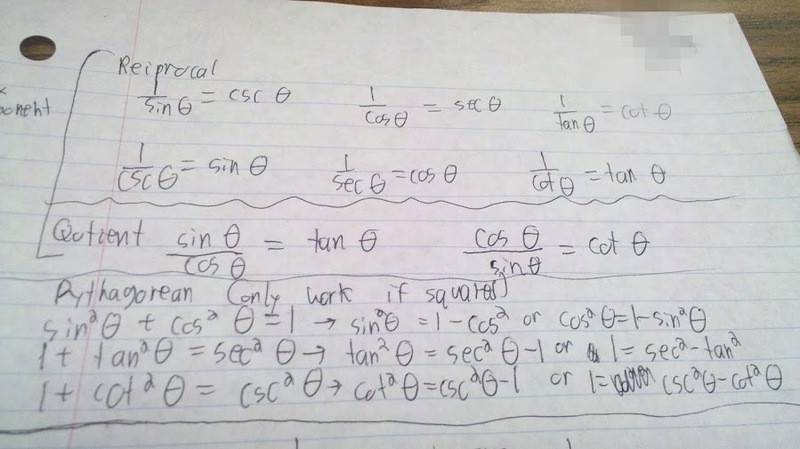# Identities sin, cos, tan etc. stuff

## Homework Statement

((cos x)/(1+sin x))+((1+sin x)/(cos x))

## Homework Equations## The Attempt at a Solution

multiplied the left equation by (cos x)/(cos x) and the right fraction by (1+sin x)/(1+sin x)

get ((cosx)^2 +(1+sinx)^2) / (1+sinx) (cos x)

and I have no idea where to go from here

Last edited by a moderator:

Mark44
Mentor

## Homework Statement

((cos x)/(1+sin x))+((1+sin x)/(cos x))
What are you trying to do, simplify the expression above?

## Homework Equations## The Attempt at a Solution

multiplied the left equation by (cos x)/(cos x) and the right fraction by (1+sin x)/(1+sin x)

get ((cosx)^2 +(1+sinx)^2) / (1+sinx) (cos x)

and I have no idea where to go from here

Yes, the answer being 2 sec x

Mark44
Mentor
Expand the numerator and simplify.
((cosx)^2 +(1+sinx)^2) / (1+sinx) (cos x)

(1-sinx^2) +1 + sinx +1 - sin x

(1- sinx^2) +2 / (1+sin x)(cos x)

I don't see how that helps

Mark44
Mentor
Expand the numerator and simplify.
((cosx)^2 +(1+sinx)^2) / (1+sinx) (cos x)

(1-sinx^2) +1 + sinx +1 - sin x
The only thing you did that makes sense is replacing cos2(x) with 1 - sin2(x). I don't get what you did go go from (1 + sin(x))2 to 1 + sin(x) + 1 - sin(x).

(1- sinx^2) +2 / (1+sin x)(cos x)

I don't see how that helps

Then what simplifying is there to do?

Mark44
Mentor
Apparently I wasn't clear. (1 + sin(x))2 ≠ 1 + sin(x) + 1 - sin(x), which seems to be what you're saying.

The "simplifying" that you need to do is to expand (1 + sin(x))2 to something it is actually equal to.

Okay it equals (1+sinx)(1-sinx)
still not way to get to 2secx

Mark44
Mentor
Because that's wrong, too. (1 + sin(x))2 ≠ (1+sinx)(1-sinx), if that's what you're saying.

How do you expand (1 + x)2? This is a similar kind of problem.

Okay it equals (1+sinx)(1+sinx)
still no way to get to 2secx

Okay it equals (1+sinx)(1+sinx)

Expand the expression: apply distributivity to work out the brackets.

All right got it